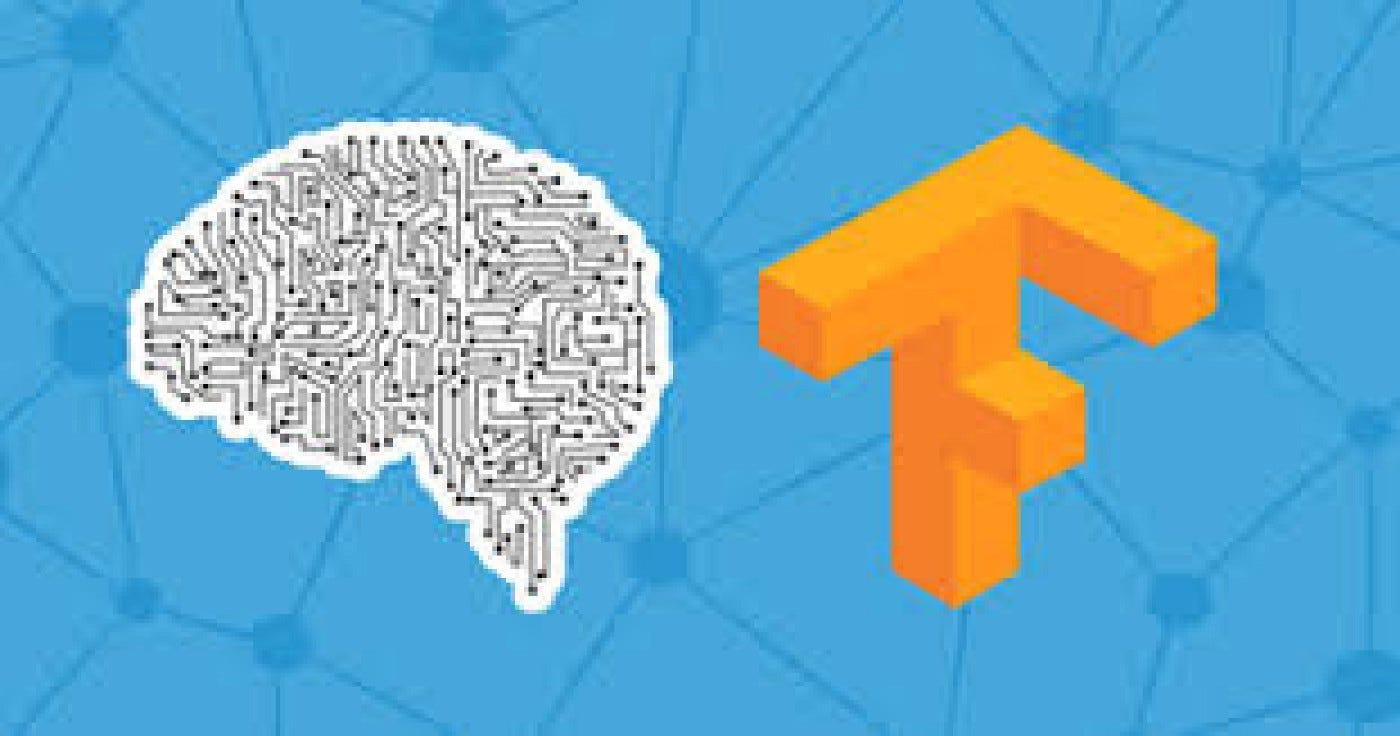# Working with Complex Image data for CNNs

Original article was published by Rishit Dagli on Deep Learning on MediumIn the previous blog post, we worked with MNIST data which was pretty simple, grayscaled 28 X 28 images, and the thing you want to classify is centered in the image. Real-life data is different, it has more complex images, your subject might be anywhere in the image not necessarily centered. Our dataset had very uniform images too. This time we’ll also work on a larger dataset.
We’ll be using the Cats vs Dogs dataset to try out these things for ourselves. TensorFlow has something called `ImageDataGenerator` which simplifies things for us and allows us to directly read the images and place them. So you would first have two directories called `train` and `validation` directory, each of the directories would have two subdirectories `Cats` and `Dogs` each of which would have the respective images and auto label them for us. Here’s how the directory structure looks-

Let’s now see this in code. The `ImageDataGenerator` is present in `tensorflow.keras.preprocessing.image` so first let’s go ahead and import it-

`from tensorflow.keras.preprocessing.image import ImageDataGenerator`

Once you do this you can now use the `ImageDataGenerator`

`train_image_generator = ImageDataGenerator(rescale=1./255)train_data_gen =  train_iamge_generator.flow_from_directory(                    batch_size=batch_size,                    directory=train_dir,                    shuffle=True,                    target_size=(IMG_HEIGHT,IMG_WIDTH)                    class_mode='binary')`

We first pass in `rescale=1./255` to normalize the images, you can then call the `flow_from_directory` the method from that directory and its sub-directories. So in this case taking the above diagram as a reference, you would pass in the `Training` directory.

Images in your data might be of different sizes to convert or resize them all into one size by the `target_size` . This is a very important step as all inputs to the neural network should be of the size. A nice thing about this code is that the images are resized for you as they’re loaded. So you don’t need to preprocess thousands of images on your file system you instead to do it in runtime.

The images will be loaded for training and validation in batches where it’s more efficient than doing it one by one. You can specify this by the `batch_size` , there are a lot of factors to consider when specifying a batch size which we will not be discussing in this blog post. But you can experiment with different sizes to see the impact on the performance.

This is a binary classifier that is it picks between two different things; cats and dogs so we specify that here by the `class_mode`.

And that’s all you need to read your data and auto label them according to their directories and also do some processing in run time. SO let’s do the same for validation data too-

`validation_image_generator = ImageDataGenerator(rescale=1./255)val_data_gen = validation_imadata_generator.flow_from_directory(                 batch_size=batch_size,                 directory= validation_dir,                 shuffle=True,                 target_size=(IMG_HEIGHT,IMG_WIDTH)                 class_mode='binary')`